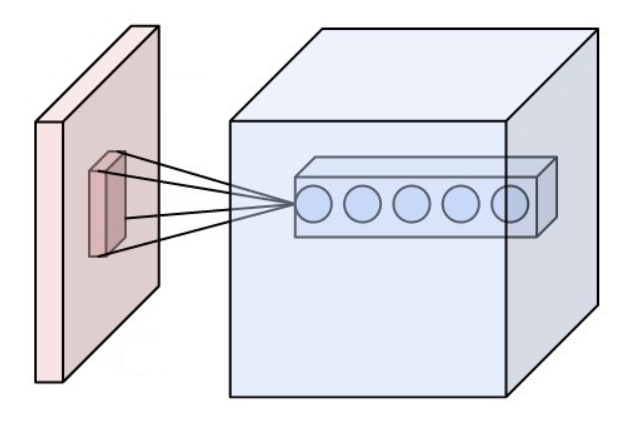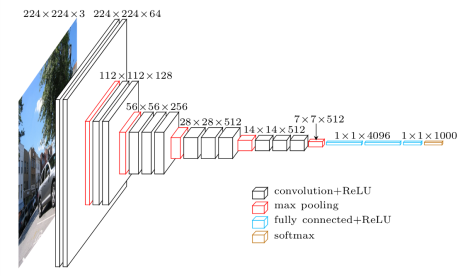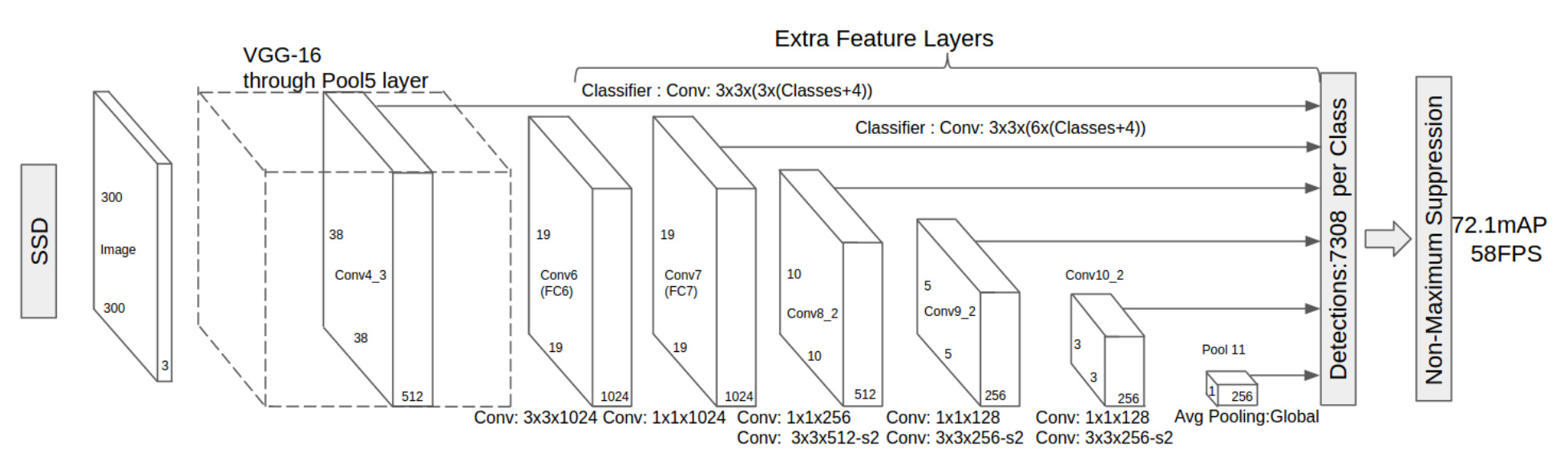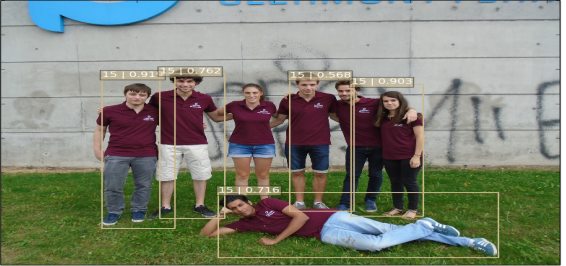Project n°4: Person detection

The goal of the project is to detect moving persons on a video using deep learning methods. We teach the neural network to detect persons on images by learning from an annotated database. More specifically we will use the SSD method thanks to the tensorflow module on python.

I Convolutionnal networks.

This method uses convolutional neural networks, that is to say neural networks that have convolutional layers:

A convolutional layer is composed of packs of neurons, each pack only processes a certain window in the initial image. A retropropagation algorithm is used to modify the activation filters that each neurone applies to the window it is assigned to.

A pooling (agregation) layer is often applied to reduce the dimension of the output after a convolutional layer.

The outputs are then connected to a fully connected layer (a layer in which each neuron is connected to every output of the previous layer) to allow an overall processing of the information.Scheme of a convolutional layer (in blue) applied on an image (in pink)

This type of network is particularly relevant as far as image recognition is concerned, as it processes information at the local scale (which is ideal for border recognition for example) allowing a much faster and adapted processing.

II VGG network

The VGG network is a a multi-layer convolutionnal network that aims to predict the probability of presence of object classes in an image. Convolutional layers are applied to the inputted image, followed by a pooling layer, then convolutional layers are applied again and so on.

After several iterations, each reducing the dimension of the output, fully connected layers are applied and finaly a classification layer gives the output probability for each class of object.Scheme of a VGG network

This model of network is one of the most efficient for image recognition, it managed to attain more than 92 % of successful recognition on the image net database.

III SSD network

The SSD network, standing for Single Shot multibox Detector, it is a method for detecting objects in an image using a single deep neural network. It's part of the family of networks which predict the bounding boxes of objects in a given image.

It is a simple, end to end single network, removing many steps involved in other networks like faster RCNN which try to achieve the same task.

The SSD network uses the VGG architecture as a base. But instead of trying to classify the image after in went throught the VGG, we make the output pass throught several other convolutionnal layers and connect the output of each layer to the final fully connected layer.A SSD scheme

As the pooling layers reduce the dimension of the image at each step, we get a processing for different sizes of the image, allowing a classification at many scales at the same time.

Unlike fasterRCNN, the SSD network doesn’t have to separate the localisation process from the classification process allowing a much faster processing .

IV Code

In the code we import ssd_vgg_300 which contain the method of a vgg, a deep neural network .

It consists in a convolutional network with filters of decreasing size so here we have :

6 layers of size (38*38, 19*19, 10*10, 5*5, 3*3 and 1*1).

Then we set the default parameters of SSD :

-im_shape defines the size of the image,

-num_classes the number of different classes that we want to use to classify the elements found in the image,

-The annotation of the label.

-The layers and the shapes.

-Anchors which are points in the image used to create boxes in order to detect some interests points, they act as filters.

-The dropout which is a value that indicates the number of neurons we keep after each step.

Now that we have the default parameters for the code, we define the specific paramters layers, the bbox (thanks to the anchor)

We need to input :

-An image.

-The number of different classes.

-The layers define before.

-The anchor_sizes and ratio defined before in the ssd_anchors_all_layers.

-The normalisation define with the boxes, and then if the net is training or not.

-The dropout.

-The function used to predict the next position of the persons/objects in the image the step after (it creates different possibilities of paths for the next step).

V Test of the network

The demo folder contains a set of images for testing the SSD algorithm in the main file. The notebook folder contains a minimal example of the SSD TensorFlow pipeline. Basically, the detection process is composed of two main steps:

1)Running the SSD network on the image

2)Post-processing the output (putting a rectangle on the detected object with a number which corresponds to the class the object belongs to).

The training data used in this SSD model is VOC datasets (2007 and 2012).
We test the algorithm with the my_test.py file, by importing the VVG neural network from the nets folder and providing the path of an image in the demo folder using the commands below:

path = './demo/'
image_names = sorted(os.listdir(path))
Example:Of the seven people present in the photograph, 5 are well recognized but 2 persons are missing.

It seems that the code can be improved to obtain better results.

Conclusion:

For this project we will use the SSD architecture, which is faster than R-CNN.

The final objective of this project is to improve the code of ssd in order to classify elements in a video which is nothing but a sequence of image.